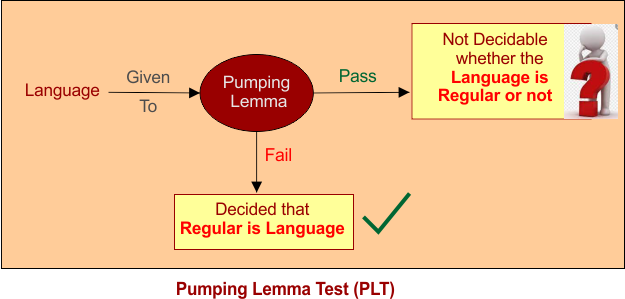Select Page

# Pumping Lemma for Regular Grammars

 V.Important Keep in mind all finite languages are regular and no need to check them whether they are regular or not but all infinite languages are not Regular languages as an,bn  is an infinite language but not a regular language.

## Pumping Lemma

Pumping Lemma is applied on infinite languages to show that languages are not regular. It should never be assure that a language is regular.## Conditions of Pumping Lemma

There are three conditions form pumping lemma. Suppose an infinite language (L) with string W = anb2n. See in string W, the length of string is greater than or equal to “n”. “n” is a pumping length.

Let divide the string into three parts i.e. w = xyz. Pumping length conditions are given below

• For all i ≥ 0, the string xyiz is also in L. here i is used to pump “y” value
• |y| > 0
• |xy| ≤ c

If the string (w = xyz) fail any condition then the string will declare itself a non-regular language.

We can say

• If given infinite Language (L)does not satisfy all three conditions of Pumping Lemma then it will be non-regular language.
• If given infinite Language satisfies all three conditions of Pumping Lemma then it may or may not be a regular language.
 Tip: As Pumping lemma gives the result on failure of any condition that’s why it is also called negative test.

## Example of Pumping Lemma

Suppose a string W = anb2n which belongs to language L. see in string W, the length of string is greater than or equal to “n” . it satisfies pumping theorem now check three conditions.

Above string (W = anb2n) said that infinite language will hold double number of “b” as compare to “a”.  If n=2 then string = aabbbb

Now divide above string into 3 parts

• X= a (first “a” from string, any part from string can be given to X, Y, Z)
• Y= abbb ( second, third, fourth and fifth)
• Z=b (last value)

Now according to first condition consider the value of i=2 which will generate the following result

W= a(abbbabbbb)b          // y pump to double

Now check pump string which is not belong to language because the original string was “string (W = anb2n) said that infinite language will hold double number of “b” as compare to “a”.

As first condition fail, so it is not a regular language.

Help Other’s By Sharing…